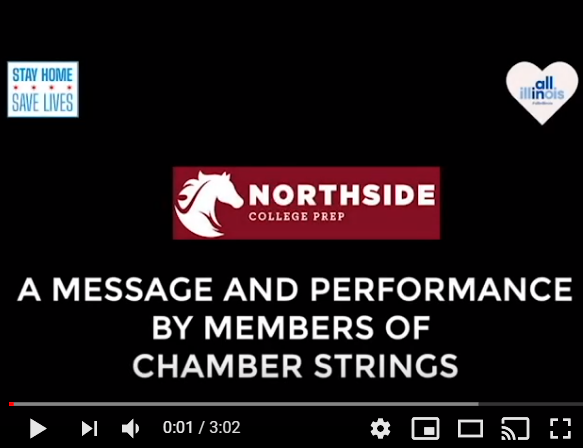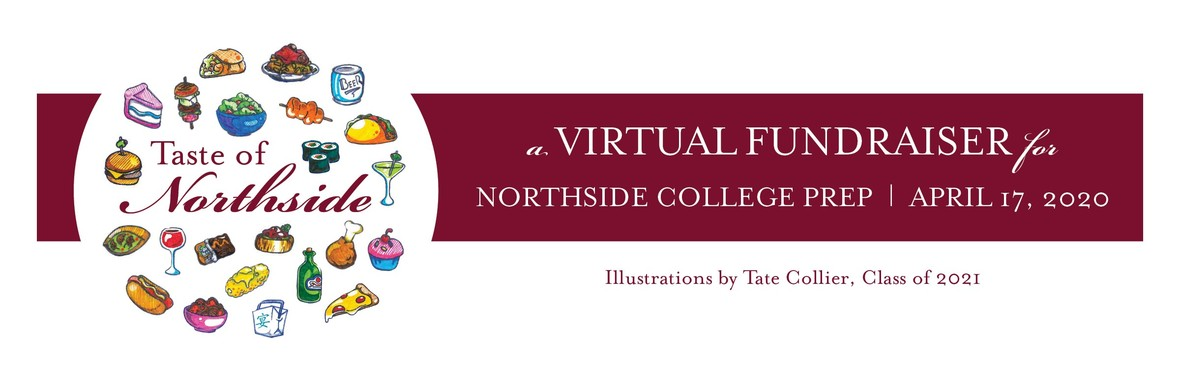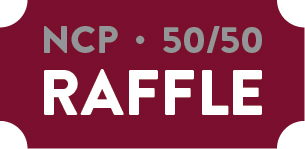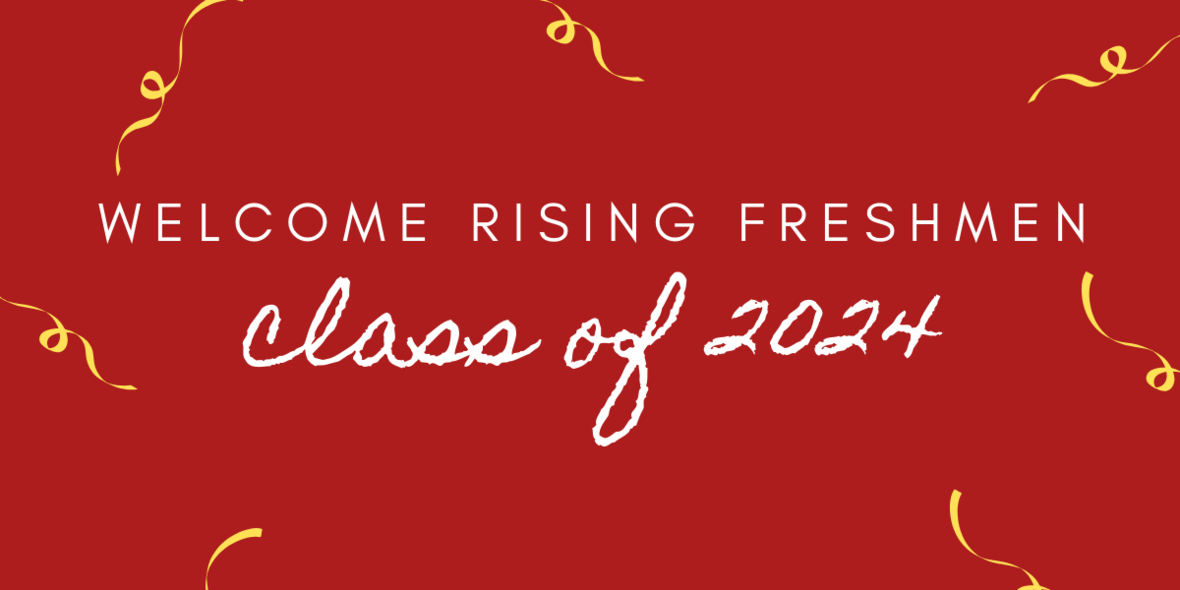# Parent Teacher Conferences This Thursday

Last week, CPS announced that Thursday, April 23 is still designated for parent-teacher conferences, as originally outlined on the CPS calendar. Students will be on a four-day schedule this week with direct engagement on Monday, Tuesday, Wednesday, and Friday.

Teachers will hold virtual office hours on Thursday for parent-teacher conferences. NCP will share additional information at the beginning of this week.

 table div table+table+table div table{width:100%;padding:0}table div table+table+table div table img{width:96.23%;padding:0;float:none}table div table+table+table div table td{width:100%;padding:0 1.88% 18px}/* styles */# A Message and Performance from NCP's Chamber StringsClick the image for a message and a performance by members of NCP's Chamber Strings.
 table div table+table+table+table+table+table div table{width:100%;padding:0}table div table+table+table+table+table+table div table img{width:96.23%;padding:0;float:none}table div table+table+table+table+table+table div table td{width:100%;padding:0 1.88% 18px}/* styles */# Raffle Drawing Winner

Congratulations to Lee Ellen Weihing, parent of freshman Nate Weihing, on winning the 2020 Taste of Northside Benefit 50/50 Raffle! Lee Ellen won half of the total proceeds of \$6,275.00, making her prize \$3,137.50.

Thanks to all who purchased raffle tickets and helped raise critical funds for Northside!

# Please Continue to Support the Paddle Raise to Build a New Turf Field

This year’s Paddle Raise is dedicated to raising funds for a much-needed, new turf athletic field. Every year, more gym classes and home athletic games must be cancelled because our Northside field is in such poor condition. Heavy use over the years has rendered it virtually unusable. A new turf field will allow our students, and future Northsiders, to play and exercise outside safely throughout the year. Let's give all of them a home-field advantage. Please demonstrate your strong support for this long-overdue improvement to the Northside campus by making a monetary contribution to the 2020 Paddle Raise.

Participate in the Paddle Raise for NCP's new turf field. Our Northside video club, the Mustang Reel, created THIS VIDEO to inspire your generosity. Let's all PONY UP, and then click HERE to see the impact of your contribution.

# Virtual "Live Auction" Postponed Indefinitely

As you know, due to the COVID-19 pandemic, we converted the Taste of Northside Spring Benefit into a virtual event, which we intended to host last Friday, April 17.

Given that so much is still in flux--including the economy and whether the offered sporting events and concert will take place this season--we feel we must postpone the virtual event to a later (to be determined) date when things stabilize. We hope, for countless reasons, that will be sooner rather than later.

Until then, we will sit tight and assess the evolving situation. Look for future announcements regarding the virtual event here, in the eblast, and on Facebook. As always, we sincerely thank you for your continued support and patience.

Thank you to those of you who have contacted Kristen Werries Collier to coordinate picking up the silent auction items that you won. If you have not done so, please email her at kristenwerriescollier@gmail.com.

Pickups are taking place in a safe, social-distancing manner from the front porch of her Wicker Park home. For those of you who do not want to venture out, Kristen will continue to hold your items and future arrangements will be made.

 /* styles */ To pay any remaining open balance, please go to Northside.GiveSmart.com. Thank you for your support!
 /* styles */ Questions? Email Debra Swan, Event Chair at debra@theswans.net.
 table div table+table+table+table+table+table+table+table+table+table+table+table+table+table+table+table div table{width:100%;padding:0}table div table+table+table+table+table+table+table+table+table+table+table+table+table+table+table+table div table img{width:96.23%;padding:0;float:none}table div table+table+table+table+table+table+table+table+table+table+table+table+table+table+table+table div table td{width:100%;padding:0 1.88% 18px}/* styles */# One Summer Chicago Application Is Open!

## Summer Jobs Available for Students - Apply Online by May 20

One Summer Chicago brings together government institutions, community-based organizations, and companies to offer over 30,000 employment and internship opportunities
to youth and young adults ages 14 to 24.

Students can apply HERE.

 table div table+table+table+table+table+table+table+table+table+table+table+table+table+table+table+table+table+table+table div table{width:100%;padding:0}table div table+table+table+table+table+table+table+table+table+table+table+table+table+table+table+table+table+table+table div table img{width:96.23%;padding:0;float:none}table div table+table+table+table+table+table+table+table+table+table+table+table+table+table+table+table+table+table+table div table td{width:100%;padding:0 1.88% 18px}/* styles *//* styles */ Follow Northside on Instagram: https://www.instagram.com/northsidecollegeprep/ Follow Northside's Asian club on Instagram: https://www.instagram.com/ncpaasa/
 table div table+table+table+table+table+table+table+table+table+table+table+table+table+table+table+table+table+table+table+table+table+table+table div table{width:100%;padding:0}table div table+table+table+table+table+table+table+table+table+table+table+table+table+table+table+table+table+table+table+table+table+table+table div table img{width:96.23%;padding:0;float:none}table div table+table+table+table+table+table+table+table+table+table+table+table+table+table+table+table+table+table+table+table+table+table+table div table td{width:100%;padding:0 1.88% 18px}/* styles */# Parent Questions - For Parents Only

As a reminder, this Question Form is available to submit your questions to the NCP administration. Please note that you will not receive a direct response to your question. Instead, this form will be used as a repository for parent questions. Once the admin team receives information or finds answers to these questions, they will be sent out as part of the parent updates.

 table div table+table+table+table+table+table+table+table+table+table+table+table+table+table+table+table+table+table+table+table+table+table+table+table+table div table{width:100%;padding:0}table div table+table+table+table+table+table+table+table+table+table+table+table+table+table+table+table+table+table+table+table+table+table+table+table+table div table img{width:96.23%;padding:0;float:none}table div table+table+table+table+table+table+table+table+table+table+table+table+table+table+table+table+table+table+table+table+table+table+table+table+table div table td{width:100%;padding:0 1.88% 18px}/* styles */# It's Not Too Late to Purchase Your 2019-2020 Yearbook

Yearbooks are still on sale for \$55:

1. Go to tinyurl.com/stampede2020
2. Enter the code: 4187220
3. Purchase!
 1 Go to tinyurl.com/stampede2020
 2 Enter the code: 4187220
 3 Purchase!
 table div table+table+table+table+table+table+table+table+table+table+table+table+table+table+table+table+table+table+table+table+table+table+table+table+table+table+table+table div table{width:100%;padding:0}table div table+table+table+table+table+table+table+table+table+table+table+table+table+table+table+table+table+table+table+table+table+table+table+table+table+table+table+table div table img{width:96.23%;padding:0;float:none}table div table+table+table+table+table+table+table+table+table+table+table+table+table+table+table+table+table+table+table+table+table+table+table+table+table+table+table+table div table td{width:100%;padding:0 1.88% 18px}/* styles */## Northside Track Teams Workout Together at Home

The Men's and Women's track teams have been using Zoom meetings to link up and do daily core workouts and bodyweight circuits. Essentially, these are group workouts, but they count reps together and seem to be having a lot of fun connecting at the same time.

 table div table+table+table+table+table+table+table+table+table+table+table+table+table+table+table+table+table+table+table+table+table+table+table+table+table+table+table+table+table+table div table{width:100%;padding:0}table div table+table+table+table+table+table+table+table+table+table+table+table+table+table+table+table+table+table+table+table+table+table+table+table+table+table+table+table+table+table div table img{width:96.23%;padding:0;float:none}table div table+table+table+table+table+table+table+table+table+table+table+table+table+table+table+table+table+table+table+table+table+table+table+table+table+table+table+table+table+table div table td{width:100%;padding:0 1.88% 18px}/* styles */# A Big Welcome to Parents of the 2024 NCP Freshmen!

 table div table+table+table+table+table+table+table+table+table+table+table+table+table+table+table+table+table+table+table+table+table+table+table+table+table+table+table+table+table+table+table+table+table div table{width:100%;padding:0}table div table+table+table+table+table+table+table+table+table+table+table+table+table+table+table+table+table+table+table+table+table+table+table+table+table+table+table+table+table+table+table+table+table div table img{width:96.23%;padding:0;float:none}table div table+table+table+table+table+table+table+table+table+table+table+table+table+table+table+table+table+table+table+table+table+table+table+table+table+table+table+table+table+table+table+table+table div table td{width:100%;padding:0 1.88% 18px}/* styles */All these great restaurants and food and beverage providers stepped up to donate their offerings for the Benefit. Many are in our own backyard and we’d like to help see them be here, serving our communities, when our city is fully operational again. Some are operating with online orders and curbside pickup. Others are closed for the next two weeks. Please consider ordering from them or purchase a gift card for a later use. Say 'thank you from the Northside community'.

 table div table+table+table+table+table+table+table+table+table+table+table+table+table+table+table+table+table+table+table+table+table+table+table+table+table+table+table+table+table+table+table+table+table+table+table+table div table{width:100%;padding:0}table div table+table+table+table+table+table+table+table+table+table+table+table+table+table+table+table+table+table+table+table+table+table+table+table+table+table+table+table+table+table+table+table+table+table+table+table div table img{width:96.23%;padding:0;float:none}table div table+table+table+table+table+table+table+table+table+table+table+table+table+table+table+table+table+table+table+table+table+table+table+table+table+table+table+table+table+table+table+table+table+table+table+table div table td{width:100%;padding:0 1.88% 18px}/* styles */## FROM THE COUNSELING DEPARTMENT

 /* styles */ Updates from the Counseling Department • Freshmen • Sophomores • Juniors • Seniors • Senior Spring Checklist
 table div table+table+table+table+table+table+table+table+table+table+table+table+table+table+table+table+table+table+table+table+table+table+table+table+table+table+table+table+table+table+table+table+table+table+table+table+table+table+table div table{width:100%;padding:0}table div table+table+table+table+table+table+table+table+table+table+table+table+table+table+table+table+table+table+table+table+table+table+table+table+table+table+table+table+table+table+table+table+table+table+table+table+table+table+table div table img{width:96.23%;padding:0;float:none}table div table+table+table+table+table+table+table+table+table+table+table+table+table+table+table+table+table+table+table+table+table+table+table+table+table+table+table+table+table+table+table+table+table+table+table+table+table+table+table div table td{width:100%;padding:0 1.88% 18px}/* styles */table div table+table+table+table+table+table+table+table+table+table+table+table+table+table+table+table+table+table+table+table+table+table+table+table+table+table+table+table+table+table+table+table+table+table+table+table+table+table+table+table+table div table{width:100%;padding:0}table div table+table+table+table+table+table+table+table+table+table+table+table+table+table+table+table+table+table+table+table+table+table+table+table+table+table+table+table+table+table+table+table+table+table+table+table+table+table+table+table+table div table img{width:96.23%;padding:0;float:none}table div table+table+table+table+table+table+table+table+table+table+table+table+table+table+table+table+table+table+table+table+table+table+table+table+table+table+table+table+table+table+table+table+table+table+table+table+table+table+table+table+table div table td{width:100%;padding:0 1.88% 18px}/* styles */